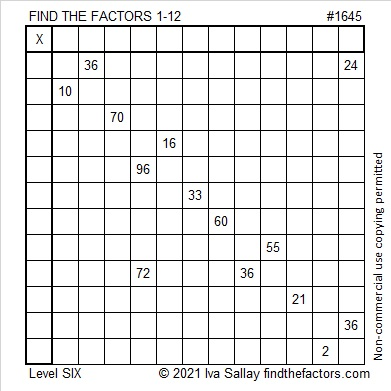# 1645 and Level 6

Contents

### Today’s Puzzle:

The number 36 appears as a clue in this puzzle three times. None of those 36’s will be 3 × 12 because 21 and 33 must use both 3’s. That means two of the 36’s will be 4 × 9, and one of them will be 6 × 6. Can both of the 36’s associated with the 24 be 4 × 9? Answering that question will help you find the logic needed to know which common factor to use for 72 and 36.### Factors of 1645:

• 1645 is a composite number.
• Prime factorization: 1645 = 5 × 7 × 47.
• 1645 has no exponents greater than 1 in its prime factorization, so √1645 cannot be simplified.
• The exponents in the prime factorization are 1, 1, and 1. Adding one to each exponent and multiplying we get (1 + 1)(1 + 1)(1 + 1) = 2 × 2 × 2 = 8. Therefore 1645 has exactly 8 factors.
• The factors of 1645 are outlined with their factor pair partners in the graphic below.### More About the Number 1645:

1645 is the hypotenuse of a Pythagorean triple:
987-1316-1645, which is (3-4-5) times 329.

This site uses Akismet to reduce spam. Learn how your comment data is processed.